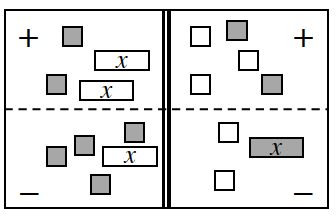### Home > CCAA8 > Chapter 3 Unit 4 > Lesson CCA: 3.2.1 > Problem3-40

3-40.

Translate the Equation Mat at right into an equation. Do not simplify your equation. Remember that the double line represents “equals.”

Count up the number of each type of tile in each section, keeping track of the tiles' signs and whether they're being added or subtracted.

$2+(−2x)−(−x+4)=−3+2−(x−2)$